Missed TensorFlow World? Check out the recap.

# CycleGAN

This notebook demonstrates unpaired image to image translation using conditional GAN's, as described in Unpaired Image-to-Image Translation using Cycle-Consistent Adversarial Networks, also known as CycleGAN. The paper proposes a method that can capture the characteristics of one image domain and figure out how these characteristics could be translated into another image domain, all in the absence of any paired training examples.

This notebook assumes you are familiar with Pix2Pix, which you can learn about in the Pix2Pix tutorial. The code for CycleGAN is similar, the main difference is an additional loss function, and the use of unpaired training data.

CycleGAN uses a cycle consistency loss to enable training without the need for paired data. In other words, it can translate from one domain to another without a one-to-one mapping between the source and target domain.

This opens up the possibility to do a lot of interesting tasks like photo-enhancement, image colorization, style transfer, etc. All you need is the source and the target dataset (which is simply a directory of images).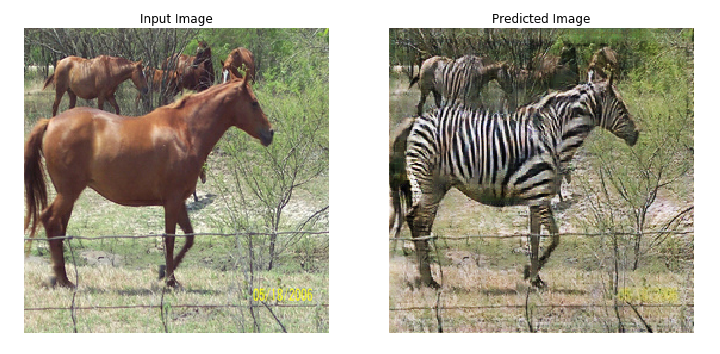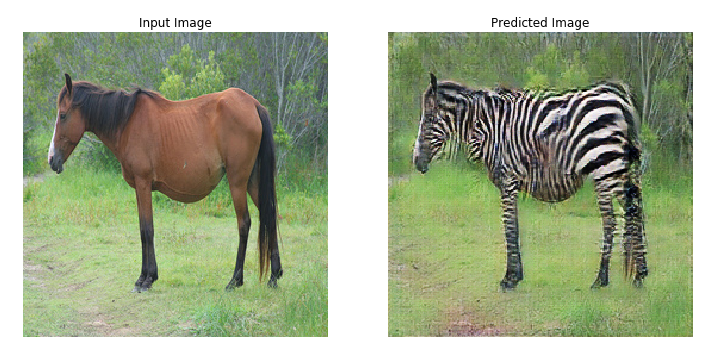## Set up the input pipeline

Install the tensorflow_examples package that enables importing of the generator and the discriminator.

!pip install -q git+https://github.com/tensorflow/examples.git

import tensorflow as tf

from __future__ import absolute_import, division, print_function, unicode_literals

import tensorflow_datasets as tfds
from tensorflow_examples.models.pix2pix import pix2pix

import os
import time
import matplotlib.pyplot as plt
from IPython.display import clear_output

tfds.disable_progress_bar()
AUTOTUNE = tf.data.experimental.AUTOTUNE


## Input Pipeline

This tutorial trains a model to translate from images of horses, to images of zebras. You can find this dataset and similar ones here.

As mentioned in the paper, apply random jittering and mirroring to the training dataset. These are some of the image augmentation techniques that avoids overfitting.

This is similar to what was done in pix2pix

• In random jittering, the image is resized to 286 x 286 and then randomly cropped to 256 x 256.
• In random mirroring, the image is randomly flipped horizontally i.e left to right.
dataset, metadata = tfds.load('cycle_gan/horse2zebra',
with_info=True, as_supervised=True)

train_horses, train_zebras = dataset['trainA'], dataset['trainB']
test_horses, test_zebras = dataset['testA'], dataset['testB']

Downloading and preparing dataset cycle_gan (111.45 MiB) to /home/kbuilder/tensorflow_datasets/cycle_gan/horse2zebra/0.1.0...

InsecureRequestWarning,

WARNING:tensorflow:From /home/kbuilder/.local/lib/python3.5/site-packages/tensorflow_datasets/core/file_format_adapter.py:209: tf_record_iterator (from tensorflow.python.lib.io.tf_record) is deprecated and will be removed in a future version.
Instructions for updating:
Use eager execution and:
tf.data.TFRecordDataset(path)

WARNING:tensorflow:From /home/kbuilder/.local/lib/python3.5/site-packages/tensorflow_datasets/core/file_format_adapter.py:209: tf_record_iterator (from tensorflow.python.lib.io.tf_record) is deprecated and will be removed in a future version.
Instructions for updating:
Use eager execution and:
tf.data.TFRecordDataset(path)

Dataset cycle_gan downloaded and prepared to /home/kbuilder/tensorflow_datasets/cycle_gan/horse2zebra/0.1.0. Subsequent calls will reuse this data.

BUFFER_SIZE = 1000
BATCH_SIZE = 1
IMG_WIDTH = 256
IMG_HEIGHT = 256

def random_crop(image):
cropped_image = tf.image.random_crop(
image, size=[IMG_HEIGHT, IMG_WIDTH, 3])

return cropped_image

# normalizing the images to [-1, 1]
def normalize(image):
image = tf.cast(image, tf.float32)
image = (image / 127.5) - 1
return image

def random_jitter(image):
# resizing to 286 x 286 x 3
image = tf.image.resize(image, [286, 286],
method=tf.image.ResizeMethod.NEAREST_NEIGHBOR)

# randomly cropping to 256 x 256 x 3
image = random_crop(image)

# random mirroring
image = tf.image.random_flip_left_right(image)

return image

def preprocess_image_train(image, label):
image = random_jitter(image)
image = normalize(image)
return image

def preprocess_image_test(image, label):
image = normalize(image)
return image

train_horses = train_horses.map(
preprocess_image_train, num_parallel_calls=AUTOTUNE).cache().shuffle(
BUFFER_SIZE).batch(1)

train_zebras = train_zebras.map(
preprocess_image_train, num_parallel_calls=AUTOTUNE).cache().shuffle(
BUFFER_SIZE).batch(1)

test_horses = test_horses.map(
preprocess_image_test, num_parallel_calls=AUTOTUNE).cache().shuffle(
BUFFER_SIZE).batch(1)

test_zebras = test_zebras.map(
preprocess_image_test, num_parallel_calls=AUTOTUNE).cache().shuffle(
BUFFER_SIZE).batch(1)

sample_horse = next(iter(train_horses))
sample_zebra = next(iter(train_zebras))

plt.subplot(121)
plt.title('Horse')
plt.imshow(sample_horse * 0.5 + 0.5)

plt.subplot(122)
plt.title('Horse with random jitter')
plt.imshow(random_jitter(sample_horse) * 0.5 + 0.5)

<matplotlib.image.AxesImage at 0x7fed182e1cf8>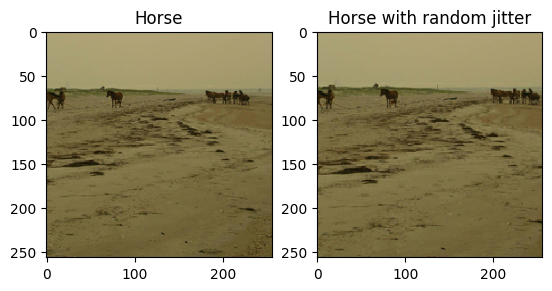plt.subplot(121)
plt.title('Zebra')
plt.imshow(sample_zebra * 0.5 + 0.5)

plt.subplot(122)
plt.title('Zebra with random jitter')
plt.imshow(random_jitter(sample_zebra) * 0.5 + 0.5)

<matplotlib.image.AxesImage at 0x7fed182be7f0>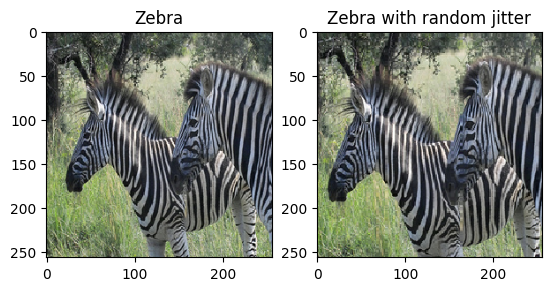## Import and reuse the Pix2Pix models

Import the generator and the discriminator used in Pix2Pix via the installed tensorflow_examples package.

The model architecture used in this tutorial is very similar to what was used in pix2pix. Some of the differences are:

There are 2 generators (G and F) and 2 discriminators (X and Y) being trained here.

• Generator G learns to transform image X to image Y. $(G: X -> Y)$
• Generator F learns to transform image Y to image X. $(F: Y -> X)$
• Discriminator D_X learns to differentiate between image X and generated image X (F(Y)).
• Discriminator D_Y learns to differentiate between image Y and generated image Y (G(X)).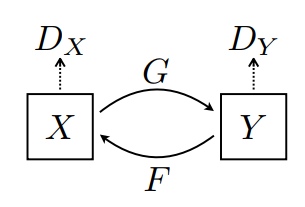OUTPUT_CHANNELS = 3

generator_g = pix2pix.unet_generator(OUTPUT_CHANNELS, norm_type='instancenorm')
generator_f = pix2pix.unet_generator(OUTPUT_CHANNELS, norm_type='instancenorm')

discriminator_x = pix2pix.discriminator(norm_type='instancenorm', target=False)
discriminator_y = pix2pix.discriminator(norm_type='instancenorm', target=False)

to_zebra = generator_g(sample_horse)
to_horse = generator_f(sample_zebra)
plt.figure(figsize=(8, 8))
contrast = 8

imgs = [sample_horse, to_zebra, sample_zebra, to_horse]
title = ['Horse', 'To Zebra', 'Zebra', 'To Horse']

for i in range(len(imgs)):
plt.subplot(2, 2, i+1)
plt.title(title[i])
if i % 2 == 0:
plt.imshow(imgs[i] * 0.5 + 0.5)
else:
plt.imshow(imgs[i] * 0.5 * contrast + 0.5)
plt.show()

WARNING:matplotlib.image:Clipping input data to the valid range for imshow with RGB data ([0..1] for floats or [0..255] for integers).
WARNING:matplotlib.image:Clipping input data to the valid range for imshow with RGB data ([0..1] for floats or [0..255] for integers).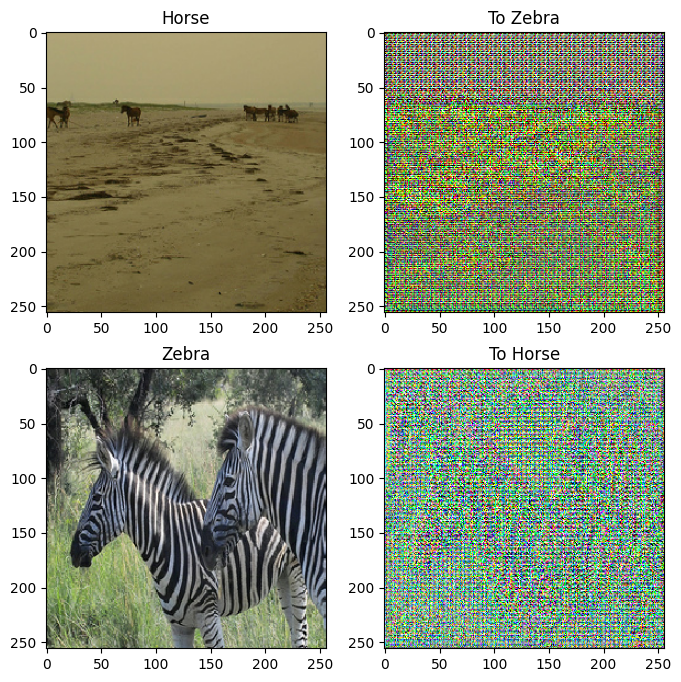plt.figure(figsize=(8, 8))

plt.subplot(121)
plt.title('Is a real zebra?')
plt.imshow(discriminator_y(sample_zebra)[0, ..., -1], cmap='RdBu_r')

plt.subplot(122)
plt.title('Is a real horse?')
plt.imshow(discriminator_x(sample_horse)[0, ..., -1], cmap='RdBu_r')

plt.show()## Loss functions

In CycleGAN, there is no paired data to train on, hence there is no guarantee that the input x and the target y pair are meaningful during training. Thus in order to enforce that the network learns the correct mapping, the authors propose the cycle consistency loss.

The discriminator loss and the generator loss are similar to the ones used in pix2pix.

LAMBDA = 10

loss_obj = tf.keras.losses.BinaryCrossentropy(from_logits=True)

def discriminator_loss(real, generated):
real_loss = loss_obj(tf.ones_like(real), real)

generated_loss = loss_obj(tf.zeros_like(generated), generated)

total_disc_loss = real_loss + generated_loss


def generator_loss(generated):
return loss_obj(tf.ones_like(generated), generated)


Cycle consistency means the result should be close to the original input. For example, if one translates a sentence from English to French, and then translates it back from French to English, then the resulting sentence should be the same as the original sentence.

In cycle consistency loss,

• Image $X$ is passed via generator $G$ that yields generated image $\hat{Y}$.
• Generated image $\hat{Y}$ is passed via generator $F$ that yields cycled image $\hat{X}$.
• Mean absolute error is calculated between $X$ and $\hat{X}$.
$$forward\ cycle\ consistency\ loss: X -> G(X) -> F(G(X)) \sim \hat{X}$$
$$backward\ cycle\ consistency\ loss: Y -> F(Y) -> G(F(Y)) \sim \hat{Y}$$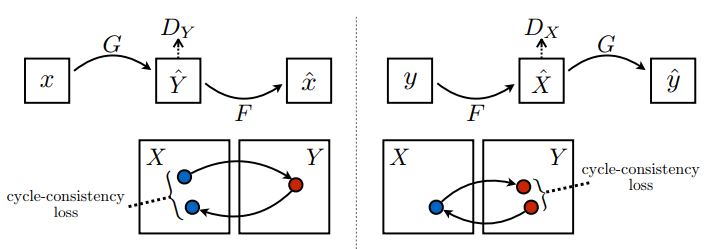def calc_cycle_loss(real_image, cycled_image):
loss1 = tf.reduce_mean(tf.abs(real_image - cycled_image))

return LAMBDA * loss1


As shown above, generator $G$ is responsible for translating image $X$ to image $Y$. Identity loss says that, if you fed image $Y$ to generator $G$, it should yield the real image $Y$ or something close to image $Y$.

$$Identity\ loss = |G(Y) - Y| + |F(X) - X|$$
def identity_loss(real_image, same_image):
loss = tf.reduce_mean(tf.abs(real_image - same_image))
return LAMBDA * 0.5 * loss


Initialize the optimizers for all the generators and the discriminators.

generator_g_optimizer = tf.keras.optimizers.Adam(2e-4, beta_1=0.5)



## Checkpoints

checkpoint_path = "./checkpoints/train"

ckpt = tf.train.Checkpoint(generator_g=generator_g,
generator_f=generator_f,
discriminator_x=discriminator_x,
discriminator_y=discriminator_y,
generator_g_optimizer=generator_g_optimizer,
generator_f_optimizer=generator_f_optimizer,
discriminator_x_optimizer=discriminator_x_optimizer,
discriminator_y_optimizer=discriminator_y_optimizer)

ckpt_manager = tf.train.CheckpointManager(ckpt, checkpoint_path, max_to_keep=5)

# if a checkpoint exists, restore the latest checkpoint.
if ckpt_manager.latest_checkpoint:
ckpt.restore(ckpt_manager.latest_checkpoint)
print ('Latest checkpoint restored!!')


## Training

EPOCHS = 40

def generate_images(model, test_input):
prediction = model(test_input)

plt.figure(figsize=(12, 12))

display_list = [test_input, prediction]
title = ['Input Image', 'Predicted Image']

for i in range(2):
plt.subplot(1, 2, i+1)
plt.title(title[i])
# getting the pixel values between [0, 1] to plot it.
plt.imshow(display_list[i] * 0.5 + 0.5)
plt.axis('off')
plt.show()


Even though the training loop looks complicated, it consists of four basic steps:

• Get the predictions.
• Calculate the loss.
• Calculate the gradients using backpropagation.
• Apply the gradients to the optimizer.
@tf.function
def train_step(real_x, real_y):
# persistent is set to True because the tape is used more than
# once to calculate the gradients.
# Generator G translates X -> Y
# Generator F translates Y -> X.

fake_y = generator_g(real_x, training=True)
cycled_x = generator_f(fake_y, training=True)

fake_x = generator_f(real_y, training=True)
cycled_y = generator_g(fake_x, training=True)

# same_x and same_y are used for identity loss.
same_x = generator_f(real_x, training=True)
same_y = generator_g(real_y, training=True)

disc_real_x = discriminator_x(real_x, training=True)
disc_real_y = discriminator_y(real_y, training=True)

disc_fake_x = discriminator_x(fake_x, training=True)
disc_fake_y = discriminator_y(fake_y, training=True)

# calculate the loss
gen_g_loss = generator_loss(disc_fake_y)
gen_f_loss = generator_loss(disc_fake_x)

total_cycle_loss = calc_cycle_loss(real_x, cycled_x) + calc_cycle_loss(real_y, cycled_y)

# Total generator loss = adversarial loss + cycle loss
total_gen_g_loss = gen_g_loss + total_cycle_loss + identity_loss(real_y, same_y)
total_gen_f_loss = gen_f_loss + total_cycle_loss + identity_loss(real_x, same_x)

disc_x_loss = discriminator_loss(disc_real_x, disc_fake_x)
disc_y_loss = discriminator_loss(disc_real_y, disc_fake_y)

# Calculate the gradients for generator and discriminator
generator_g.trainable_variables)
generator_f.trainable_variables)

discriminator_x.trainable_variables)
discriminator_y.trainable_variables)

# Apply the gradients to the optimizer
generator_g.trainable_variables))

generator_f.trainable_variables))

discriminator_x.trainable_variables))

discriminator_y.trainable_variables))

for epoch in range(EPOCHS):
start = time.time()

n = 0
for image_x, image_y in tf.data.Dataset.zip((train_horses, train_zebras)):
train_step(image_x, image_y)
if n % 10 == 0:
print ('.', end='')
n+=1

clear_output(wait=True)
# Using a consistent image (sample_horse) so that the progress of the model
# is clearly visible.
generate_images(generator_g, sample_horse)

if (epoch + 1) % 5 == 0:
ckpt_save_path = ckpt_manager.save()
print ('Saving checkpoint for epoch {} at {}'.format(epoch+1,
ckpt_save_path))

print ('Time taken for epoch {} is {} sec\n'.format(epoch + 1,
time.time()-start))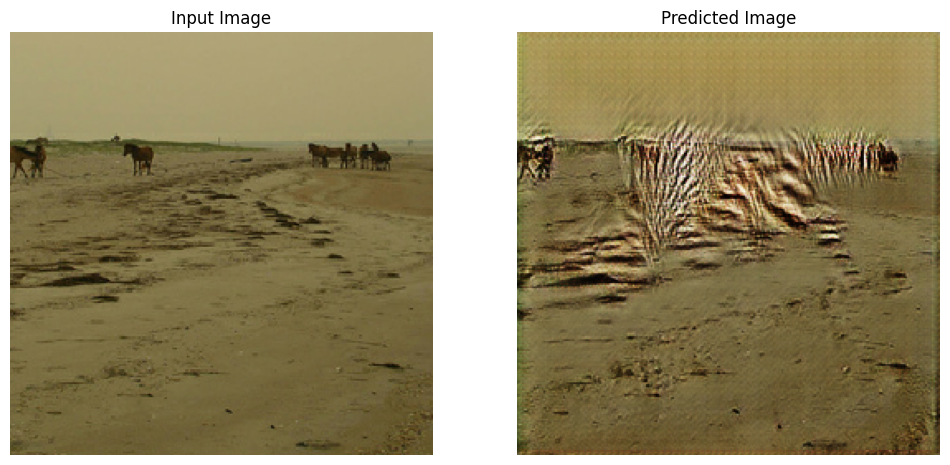Saving checkpoint for epoch 40 at ./checkpoints/train/ckpt-8
Time taken for epoch 40 is 183.51983284950256 sec



## Generate using test dataset

# Run the trained model on the test dataset
for inp in test_horses.take(5):
generate_images(generator_g, inp)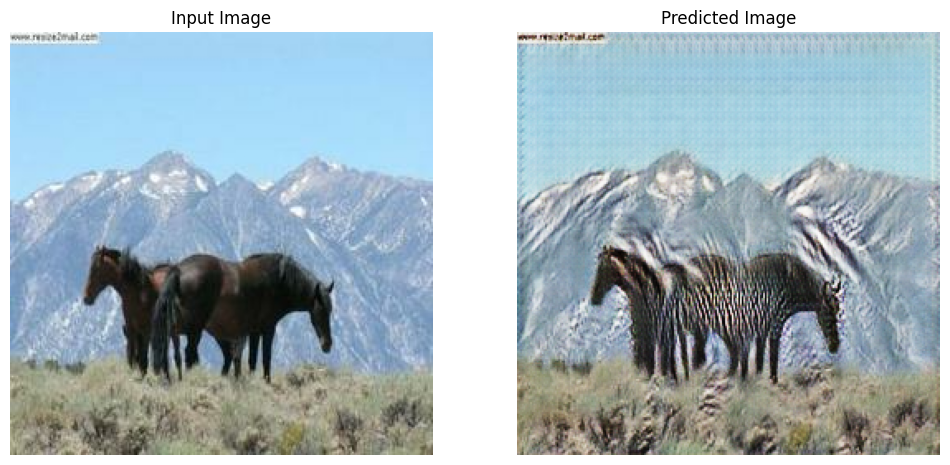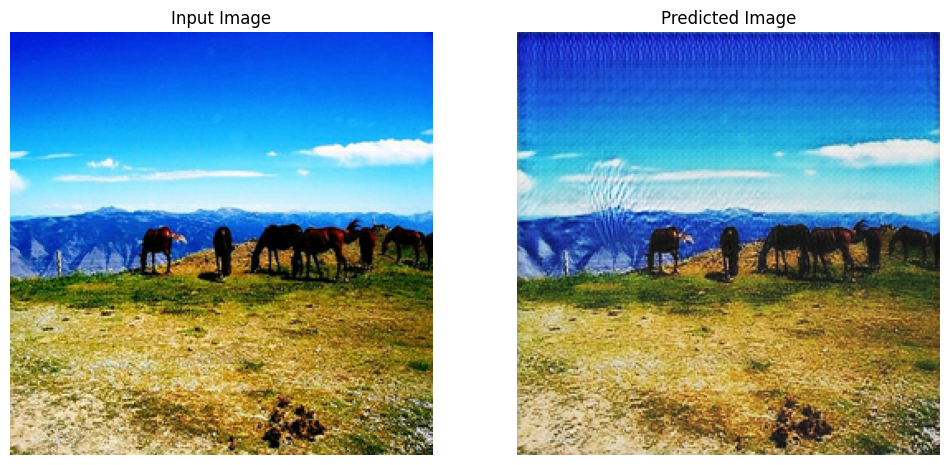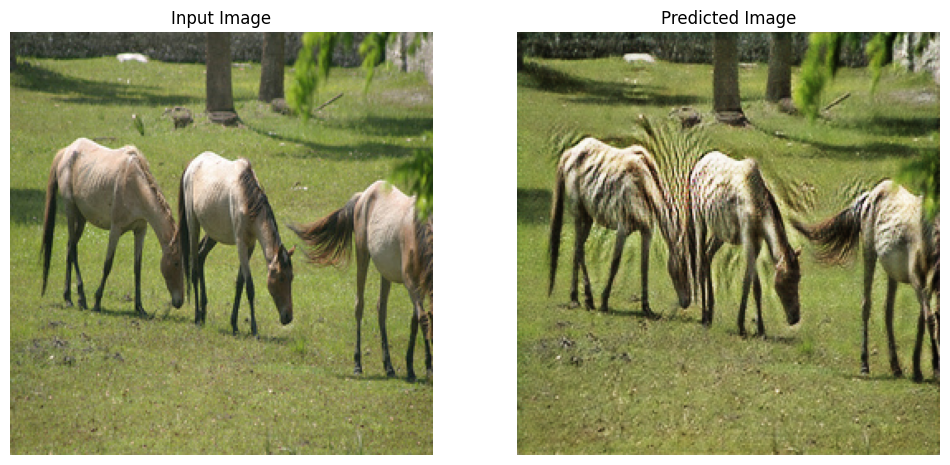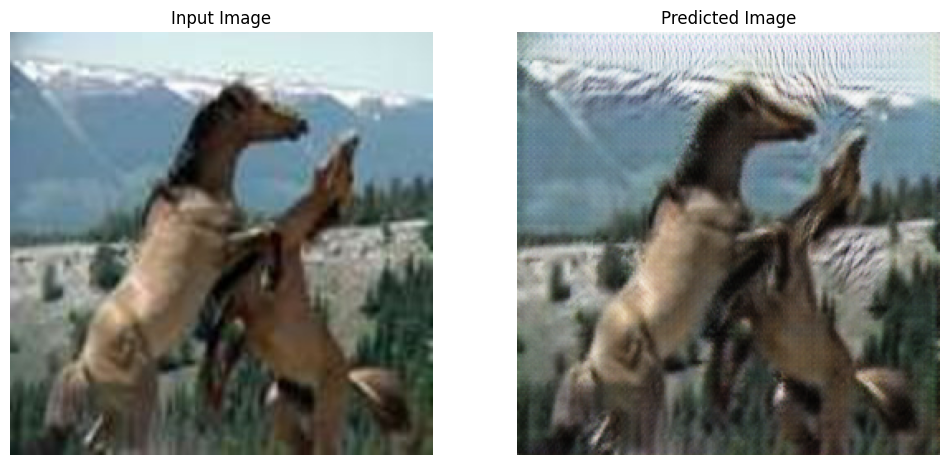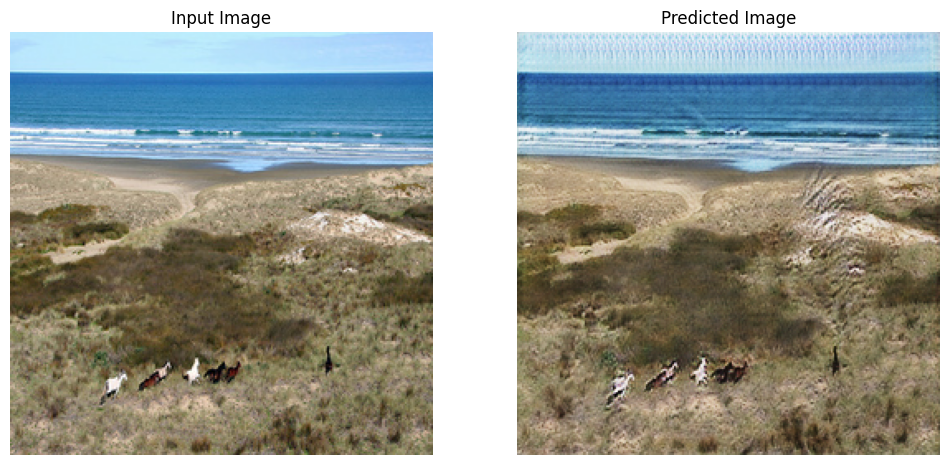## Next steps

This tutorial has shown how to implement CycleGAN starting from the generator and discriminator implemented in the Pix2Pix tutorial. As a next step, you could try using a different dataset from TensorFlow Datasets.

You could also train for a larger number of epochs to improve the results, or you could implement the modified ResNet generator used in the paper instead of the U-Net generator used here.